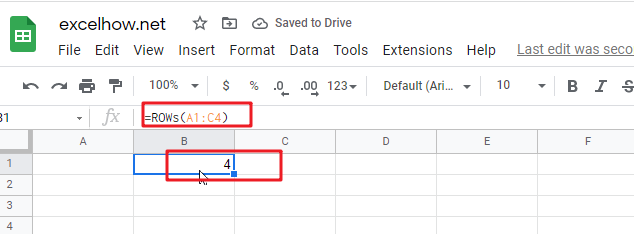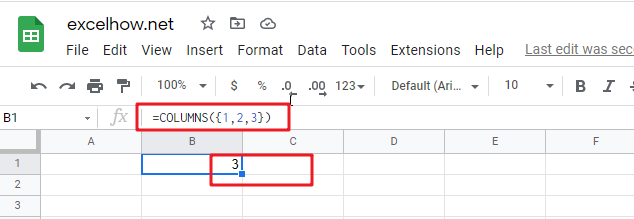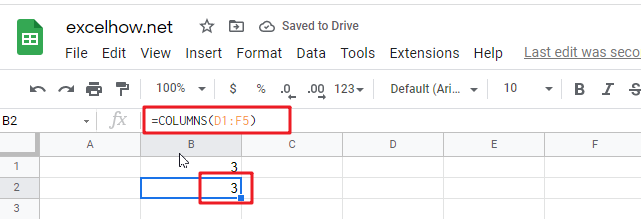This post will guide you how to use Google Sheets ROWS function with syntax and examples.

## Description

The Google Sheets ROWS function counts the number of rows in a cell reference.

The ROWS function can be used to return the number of rows in a specified range in google sheets. The purpose of this function is to get the number of rows in an array or cell reference and It’s returned value is a number of rows.

The ROWS function is a build-in function in Google Sheets and it is categorized as a LOOKUP function.

## Syntax

The syntax of the ROWS function is as below:

= ROWS(array)

Where the ROWS function arguments is:

• array-This is an required argument. An array, or A reference to a range of cells.

Note:

• ROWS just takes one argument, it can be a range or a array.
• Array can be a range or a cell reference
• If you want to count columns, you can use the COLUMNS function.
• If you want to get row numbers, you can use the ROW function.

## Google Sheets ROWS Function Examples

The below examples will show you how to use google sheets ROWS Function to return the number of rows in a range of cells.

#1 To get the number of rows in the cell range “A1:C4”, just using the following ROWS formula:

=ROWS(A1:C4)This post will guide you how to use Google Sheets COLUMNS function with syntax and examples.

## Description

The Google Sheets COLUMNS function returns the number of columns in an Array or a reference. For example, COLUMNS(B1:D4) returns 3, since the range B1:D4 contains 3 columns.

The COLUMNS function can be used to get the count of columns in a given cell range in google sheets. The purpose of this function is to get the number of columns in an array range or cell reference and the returned value is a number that representing the COLUMNS.

The COLUMNS function is a build-in function in Google Sheets and it is categorized as a Lookup function.

## Syntax

The syntax of the COLUMNS function is as below:

=COLUMNS (array)

Where the COLUMNS function arguments are:

• Array -This is a required argument. A reference to a cell or a range of cells.

Notes:

• Array can be a cell range or a reference that containing a group of cells
• If you want to get column numbers, just see also COLUMN function.
• If you want to get row numbers, just see also ROW function.
• If you want to get the number of rows in given array or cell range, just see also ROWs function.

## Google Sheets COLUMNS Function Examples

The below examples will show you how to use google sheets COLUMNS to return the number of columns in a given range.

#1 To get the number of columns in the reference D1:F5, just using the following excel formula:

`=COLUMNS(D1:F5)`#2 Using COLUMNS function to get a column count for a given array constant “{1,2,3}”, just using the following formula:

`=COLUMNS({1,2,3})`